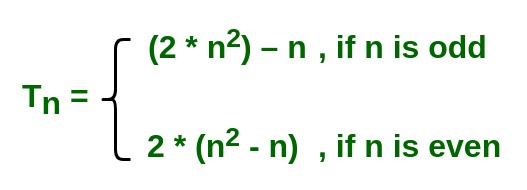# Program to find nth term of the series 1 4 15 24 45 60 92

Given a number n, the task is to find the nth term of the series

1, 4, 15, 24, 45, 60, 91, 112, 153…..

where 0 < n < 100000000.

Examples:

```Input: n = 10
Output: 180

Input: n = 5
Output: 45
```

Approach:
The idea is very simple but hard to recognise.
If n is odd, the nth term will be [ ( 2 * ( n^2 ) ) – n ].
If n is even, the nth term will be [ 2 * ( (n^2) – n ) ].Implementation:

## C++

 `#include ` ` `  `// function to calculate nth term of the series ` `long` `long` `int` `nthTerm(``long` `long` `int` `n) ` `{ ` `    ``// variable nth will store the nth term of series ` `    ``long` `long` `int` `nth; ` ` `  `    ``// if n is even ` `    ``if` `(n % 2 == 0) ` `        ``nth = 2 * ((n * n) - n); ` ` `  `    ``// if n is odd ` `    ``else` `        ``nth = (2 * n * n) - n; ` ` `  `    ``// return nth term ` `    ``return` `nth; ` `} ` ` `  `// Driver code ` `int` `main() ` `{ ` `    ``long` `long` `int` `n; ` ` `  `    ``n = 5; ` ` `  `    ``printf``(``"%lld\n"``, nthTerm(n)); ` ` `  `    ``n = 25; ` `    ``printf``(``"%lld\n"``, nthTerm(n)); ` ` `  `    ``n = 25000000; ` `    ``printf``(``"%lld\n"``, nthTerm(n)); ` ` `  `    ``n = 250000007; ` `    ``printf``(``"%lld\n"``, nthTerm(n)); ` ` `  `    ``return` `0; ` `} `

## Java

 `// Java implementation of the above approach ` ` `  `class` `GFG ` `{ ` ` `  `    ``// function to calculate nth  ` `    ``// term of the series  ` `    ``static` `long` `nthTerm(``long` `n)  ` `    ``{  ` `        ``// variable nth will store the ` `        ``// nth term of series  ` `        ``long` `nth;  ` `     `  `        ``// if n is even  ` `        ``if` `(n % ``2` `== ``0``)  ` `            ``nth = ``2` `* ((n * n) - n);  ` `     `  `        ``// if n is odd  ` `        ``else` `            ``nth = (``2` `* n * n) - n;  ` `     `  `        ``// return nth term  ` `        ``return` `nth;  ` `    ``}  ` `     `  `    ``// Driver code  ` `    ``public` `static` `void` `main(String []args)  ` `    ``{  ` `        ``long` `n;  ` `        ``n = ``5``;  ` `        ``System.out.println(nthTerm(n));  ` `     `  `        ``n = ``25``;  ` `        ``System.out.println(nthTerm(n));  ` `     `  `        ``n = ``25000000``;  ` `        ``System.out.println(nthTerm(n));  ` `     `  `        ``n = ``250000007``;  ` `        ``System.out.println(nthTerm(n));  ` `    ``}  ` `} ` ` `  `// This code is contributed by Ryuga `

## Python3

 `# function to calculate nth term of the series ` `def` `nthTerm(n): ` ` `  `    ``# variable nth will store the nth  ` `    ``# term of series ` `    ``nth ``=` `0` ` `  `    ``# if n is even ` `    ``if` `(n ``%` `2` `=``=` `0``): ` `        ``nth ``=` `2` `*` `((n ``*` `n) ``-` `n) ` ` `  `    ``# if n is odd ` `    ``else``: ` `        ``nth ``=` `(``2` `*` `n ``*` `n) ``-` `n ` ` `  `    ``# return nth term ` `    ``return` `nth ` ` `  `# Driver code ` `n ``=` `5` ` `  `print``(nthTerm(n)) ` ` `  `n ``=` `25` `print``(nthTerm(n)) ` ` `  `n ``=` `25000000` `print``(nthTerm(n)) ` ` `  `n ``=` `250000007` `print``(nthTerm(n)) ` ` `  `# This code is contributed by  ` `# Mohit kumar 29 `

## C#

 `// C# implementation of the above approach ` `using` `System; ` ` `  `class` `GFG ` `{ ` ` `  `    ``// function to calculate nth  ` `    ``// term of the series  ` `    ``static` `long` `nthTerm(``long` `n)  ` `    ``{  ` `        ``// variable nth will store the ` `        ``// nth term of series  ` `        ``long` `nth;  ` `     `  `        ``// if n is even  ` `        ``if` `(n % 2 == 0)  ` `            ``nth = 2 * ((n * n) - n);  ` `     `  `        ``// if n is odd  ` `        ``else` `            ``nth = (2 * n * n) - n;  ` `     `  `        ``// return nth term  ` `        ``return` `nth;  ` `    ``}  ` `     `  `    ``// Driver code  ` `    ``public` `static` `void` `Main()  ` `    ``{  ` `        ``long` `n;  ` `        ``n = 5;  ` `        ``Console.WriteLine(nthTerm(n));  ` `     `  `        ``n = 25;  ` `        ``Console.WriteLine(nthTerm(n));  ` `     `  `        ``n = 25000000;  ` `        ``Console.WriteLine(nthTerm(n));  ` `     `  `        ``n = 250000007;  ` `        ``Console.WriteLine(nthTerm(n));  ` `    ``}  ` `} ` ` `  `// This code is contributed by chandan_jnu `

## PHP

 ` `

Output:

```45
1225
1249999950000000
125000006750000091
```

Attention reader! Don’t stop learning now. Get hold of all the important DSA concepts with the DSA Self Paced Course at a student-friendly price and become industry ready.

My Personal Notes arrow_drop_upCheck out this Author's contributed articles.

If you like GeeksforGeeks and would like to contribute, you can also write an article using contribute.geeksforgeeks.org or mail your article to contribute@geeksforgeeks.org. See your article appearing on the GeeksforGeeks main page and help other Geeks.

Please Improve this article if you find anything incorrect by clicking on the "Improve Article" button below.